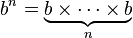## Nth Power(Exponents) Calculator

 n : b : Nth Power :

Exponentiation is a mathematical operation, written as bn, given base number b and exponent n.

### exponent calculator formula:When n is a positive integer, exponentiation corresponds to repeated multiplication.

For example:102 = 100
82 = 64
32 = 9

Thinkcalculator.com provides you helpful and handy calculator resources.